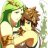# HackingBasiliskii Vita keymap with more keys

#### rsn8887

##### Well-Known Member
OP
Member
I made an extended keymapping (imap) file for Basiliskii Vita, where more keys are mapped to Vita button combinations. The download (zip) is here:
https://github.com/rsn8887/basiliskii-vita-imap-files/releases

To use, just extract the files over into the ux0:/data/basiliskii/imap folder. Then, in the running emulator, press select to open the menu, use dpad left/right to choose a keymap file, and press x to load the mapping.

Currently, there is only one mapping called "morekeys."

It is far from perfect, but pretty useful to enter a few letters or numbers, or press a key here and there.

Here are the supported mappings:
Code:
``````"morekeys" Basiliskii Vita input map extended with more keys
by rsn8887

cursor up = up
cursor right = right
cursor down = down
cursor left = left
backspace = triangle
space = circle
mouse button 0 = cross
enter = square
a = lTrigger+triangle
b = lTrigger+circle
c = lTrigger+cross
d = lTrigger+square
e = lTrigger+up
f = lTrigger+right
g = lTrigger+down
h = lTrigger+left
i = rTrigger+triangle
j = rTrigger+circle
k = rTrigger+cross
l = rTrigger+square
m = rTrigger+up
n = rTrigger+right
o = rTrigger+down
p = rTrigger+left
q = lTrigger+rTrigger+triangle
r = lTrigger+rTrigger+circle
s = lTrigger+rTrigger+cross
t = lTrigger+rTrigger+square
u = lTrigger+rTrigger+up
v = lTrigger+rTrigger+right
w = lTrigger+rTrigger+down
x = lTrigger+rTrigger+left
y = start+triangle
z = start+circle
1 = start+cross
2 = start+square
3 = start+up
4 = start+right
5 = start+down
6 = start+left
7 = lTrigger+start+triangle
8 = lTrigger+start+circle
9 = lTrigger+start+cross
0 = lTrigger+start+square
tab = lTrigger+start+up
esc = lTrigger+start+right
f1 = lTrigger+start+down
f2 = lTrigger+start+left
f3 = rTrigger+start+triangle
f4 = rTrigger+start+circle
f5 = rTrigger+start+cross
f6 = rTrigger+start+square
f7 = rTrigger+start+up
f8 = rTrigger+start+right
f9 = rTrigger+start+down
f10 = rTrigger+start+left
f11 = lTrigger+rTrigger+start+triangle
f12 = lTrigger+rTrigger+start+circle
, = lTrigger+rTrigger+start+cross
. = lTrigger+rTrigger+start+square
; = lTrigger+rTrigger+start+up
= = lTrigger+rTrigger+start+right
- = lTrigger+rTrigger+start+down
[ = lTrigger+rTrigger+start+left``````

••Replies
4
Views
2K
•Replies
2
Views
876
Replies
0
Views
1K
••Replies
36
Views
9K
•Replies
16
Views
30K

### Recent Content

9. #### ACNH Build your own Treasure island@ Sonic Angel Knight: I'm not paying over 1000 for a phone